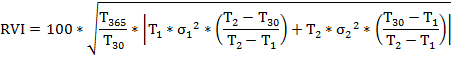# The New Russian Volatility Index – RVI

Taking into account the latest international financial industry standards, as well as, feedback from market participants, on April 16, 2014, the Moscow Exchange has officially launched the RVI – the new Russian volatility index.  Reuters code: .RVI, Bloomberg code: RVI\$ Index.

The new RVI measures market's expectation of the 30-day volatility, calculated from real prices of near- and next-series RTS Index options. In the previous RTSVX volatility index, a parameterized volatility smile was used to calculate continuous, theoretical Black-Scholes prices of the near- and next-series RTS Index options.

The RVI is calculated in real-time during, both, day and evening sessions (first values 19:00 – 23:50 MSK and then 10:00 – 18:45 MSK).

Main differences from the RTSVX volatility index:

• discrete calculation
• uses actual options prices over 15 strikes
• calculates 30-day volatility

The RVI formula:where:
Т30 — 30 days, expressed as a fraction of a year (year = 365 days);
Т365 — 365 days, expressed as a fraction of a year;
Т1 — time to expiration1, expressed as a fraction of a calendar year (year = 365 days) of the near-series options;
T2 — time to expiration1, expressed as a fraction of a calendar year (year = 365 days) of the far-series options;
σ12 — variance of the near-series options;
σ22 — variance of the next-series options.

____________________________________
1Expiration refers to the last exercise date of a given options series.

# Russian Volatility Index - RTSVX

Index calculation is discontinued on December 12, 2016

The Russian Volatility Index is an aggregated indicator that tracks the performance of the futures and options market.  The Index is calculated on the basis of volatility levels of the nearby and next series of options on RTS Index futures.

The Russian Volatility Index was introduced on 7 December 2010.

Black-Scholes option pricing formula for futures-style options with futures as the underlying asset is applied to calculate the Index's values. Option's volatility level determined based on regular Exchange's volatility curve truncated by two parameters is used for the calculation. The truncation is applied in order to single out strike ranges with stable options quotations.